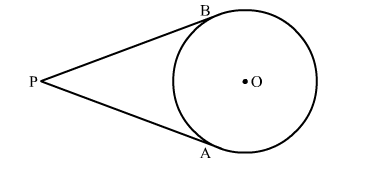# The number of tangents that can be drawn from an external point to a circle is`
Question:

The number of tangents that can be drawn from an external point to a circle is

(1) 1
(2) 2
(3) 3
(4) 4

Solution:

We can draw only two tangents from an external point to a circle.Hence, the correct answer is option (b)